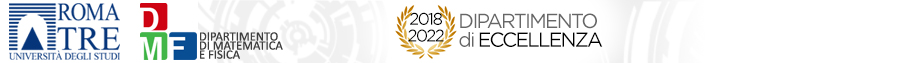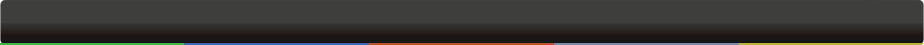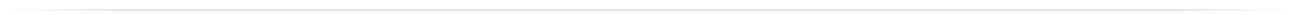# Seminari del Dipartimento

 GeometriaAbout the Severi Problem on toric surfacesLionel Lang 18-10-2018 - 14:30Largo San Leonardo Murialdo,1 - Pal.C - Aula 211 In 1986, J. Harris proved that the Severi variety  V^{d,g}:= {irreducible nodal curve of degree d and genus g in the projective plane} is irreducible for any g<(d-1)(d-2)/2. To do so, Harris proceeds in 2 steps: (1) any irreducible component of V^{d,g} contains the locus of irreducible nodal rational curves in its closure, (2) the monodromy of the space of rational curves permute all the sheets of V^{d,g}. Using the same strategy, we are interested in determining the irreducibility of the Severi variety V^{X,|L|,g}:={irreducible nodal curve of genus g in a given ample linear system |L| on a toric surface X}. In 2007, I. Tyomkin proved that V^{X,|L|,g} is irreducible for any ample linear system |L| on a Hirzebruch surface X. In 2014, he provided a pair (X, |L|) for which V^{X,|L|,1} is reducible. In this talk, we aim to show that for any toric surface X, the irreducibility of V^{X,|L|,g} depends only on the surface X provided that |L| is "big" enough. Apparently, the step (1) is already available in this generality from the work of [Caporaso-Harris, Vakil]. For step (2), we will show how one can compute the monodromy of the space V^{X,|L|,0} using rather elementary computations and a bit of combinatorics. This is a work in progress.org: VIVIANI FilippoCopyright© 2014 Dipartimento di Matematica e Fisica